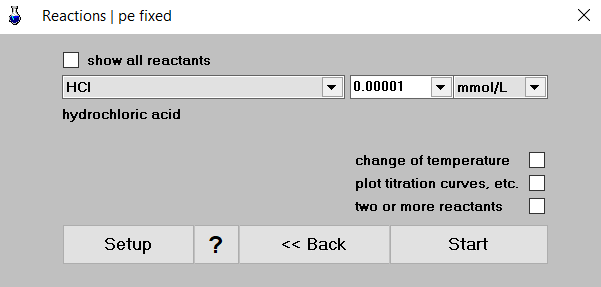# pH of an Extremely Dilute Acid

Problem

What is the pH of a 10-8 molar HCl solution? Compare the numerical result of aqion with the exact solution of the corresponding equations.

1. Numerical Solution with aqionWe begin with pure water (button H2O), then click on Reac and enter for “HCl” the value 1e-5 mmol/L – see the right screenshot. (Please mind the concentration units: 10-8 mol/L = 10-5 mmol/L.)

Start the calculation by clicking on the Start button. The result appears immediately:

 pH = 6.98

The highly diluted HCl solution has pH 6.98. This value is at least below pH 7 (which we also expect from an acid).

2. Exact Solution (Theory)

Let’s abbreviate the total HCl concentration by CT = 10-8 M. The aqueous system consists of three components: [H+], [OH-], and [Cl-]. Thus, we need three equations:

 (1.1) strong acid (completely dissociated): [Cl-] = CT (1.2) charge balance: [H+] = [OH-] + [Cl-] (1.3) self-ionization of water: Kw = [H+] [OH-] = 10-14

Inserting the first two equations into the third yields a quadratic equation in x = [H+]:

 (2) x2 – CT x – Kw = 0

The (non-negative) solution for x is

 (3) $$x \ = \ \dfrac{C_T}{2}\, \left\{ 1 + \sqrt{1+\dfrac{4K_w}{C_T^2}} \right\}$$

After inserting the numbers into the equation we get:

 (4) $$x \ = \ \dfrac{1.0\cdot 10^{-8} M}{2} \, \left( 1 + \sqrt{\strut 1+400} \ \right) \ = \ 10.51 \cdot 10^{-8} M$$

The negative decadic logarithm of x defines the pH1

 (5) pH  =  –lg [H+]  =  –lg x  =  6.978

This is in perfect agreement with the numerical result above.2

Pitfalls

We fall into a deep error if we identify the H+ concentration with that of HCl, that is, if we set [H+] = 10-8 M into the last equation:

 (6) pH  =  –lg [H+]  =  –lg 10-8  =  8 ⇐ that’s wrong

That’s definitely wrong because an acid cannot have pH > 7. Where did we make a mistake?

The answer is simple: We ignored the 10-7 mol/L H+ that comes from the self-ionization of water — see 1.3. To this background concentration of 1.0·10-7 M we should add the small amount of 0.1·10-7 M from HCl.